## The Indian coast guard, while patrolling, saw a suspicious boat with people. They were nowhere looking like fishermen. The coast guard were closely observing the movement of the boat for an opportunity to seize the boat. They observed that the boat is moving along a planar surface. At an instant of time, the coordinates of the position of the coast guard helicopter and the boat is (1, 3, 5) and (2, 5, 3) respectively.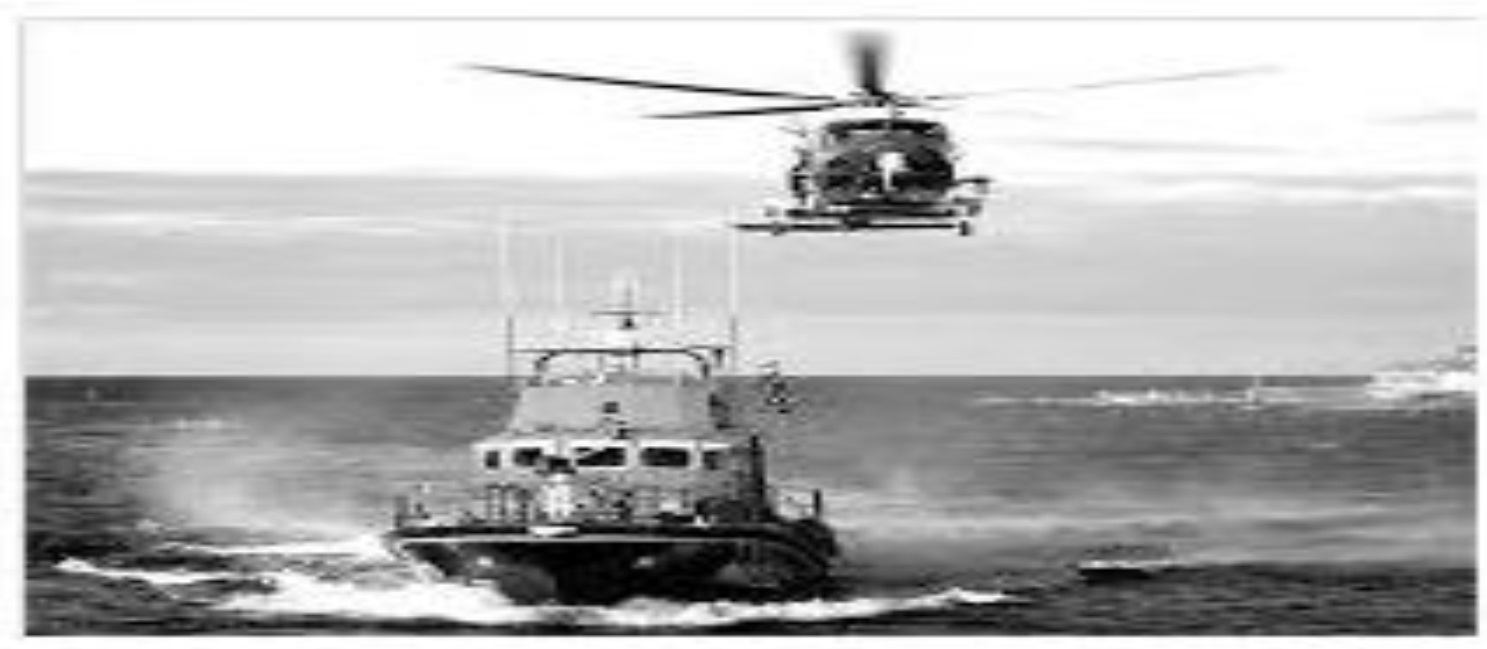(adsbygoogle = window.adsbygoogle || []).push({}); Based on the above answer the following: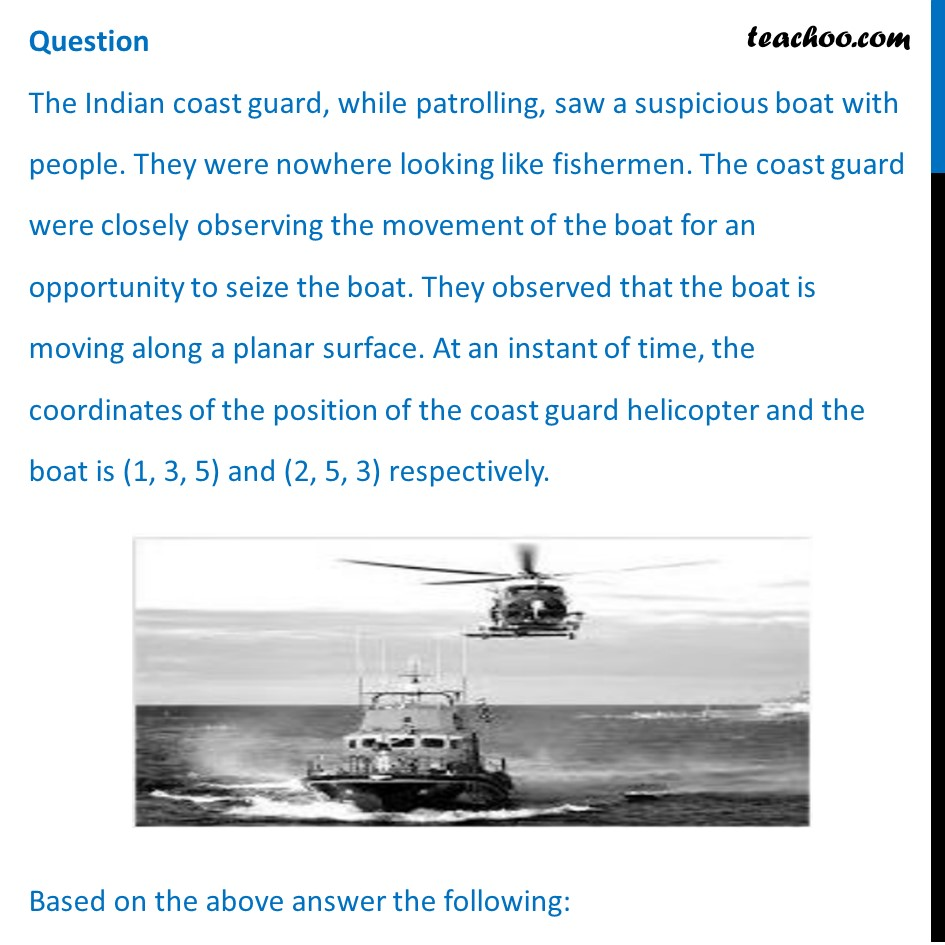## (d) x – 2y – 2z = 6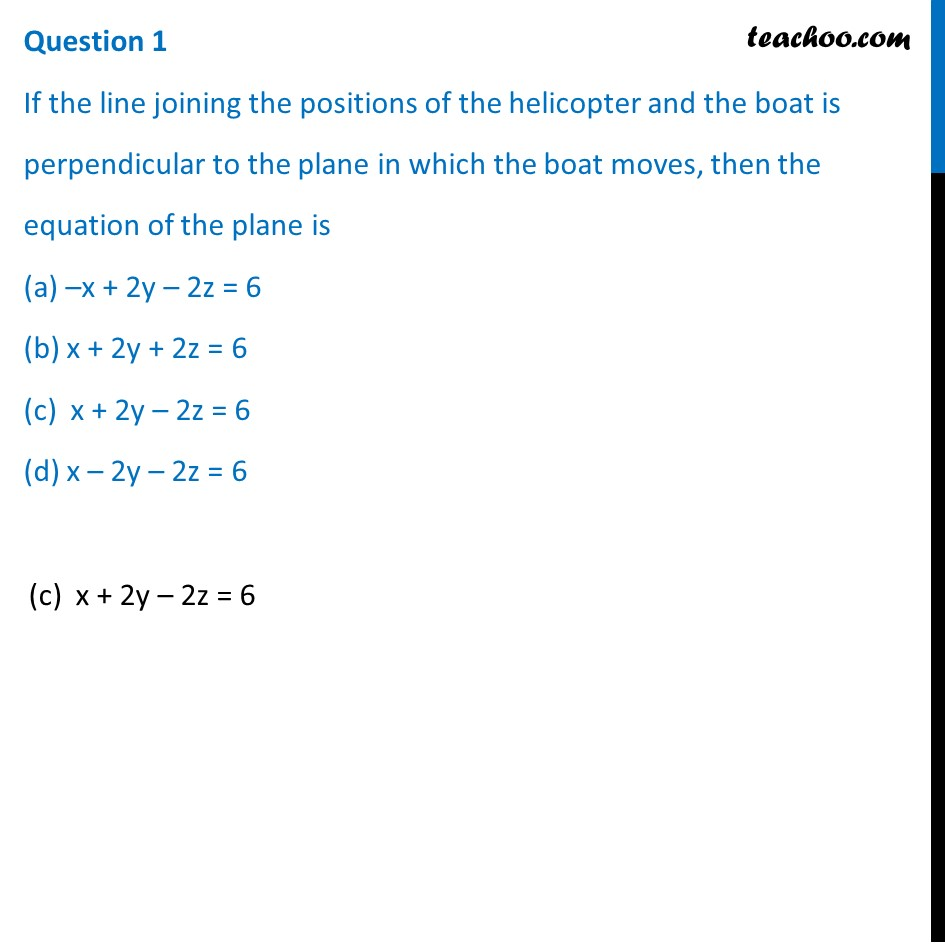## (d) 4m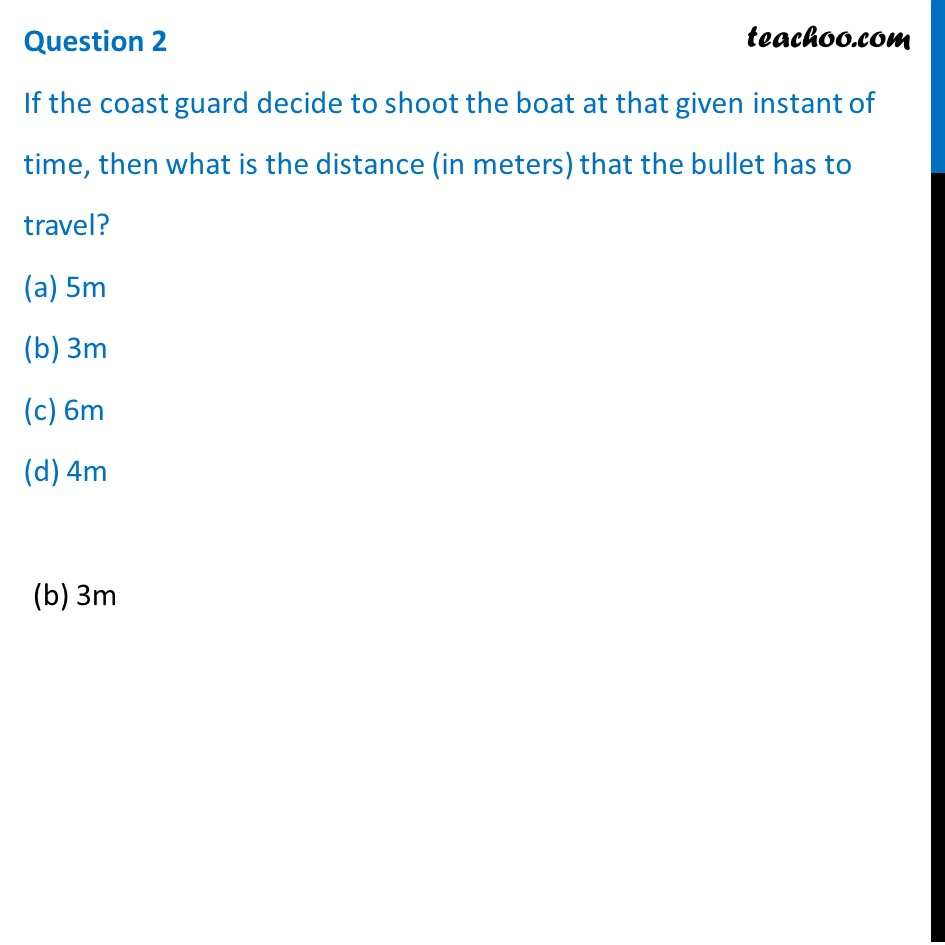## (d) 1/12 seconds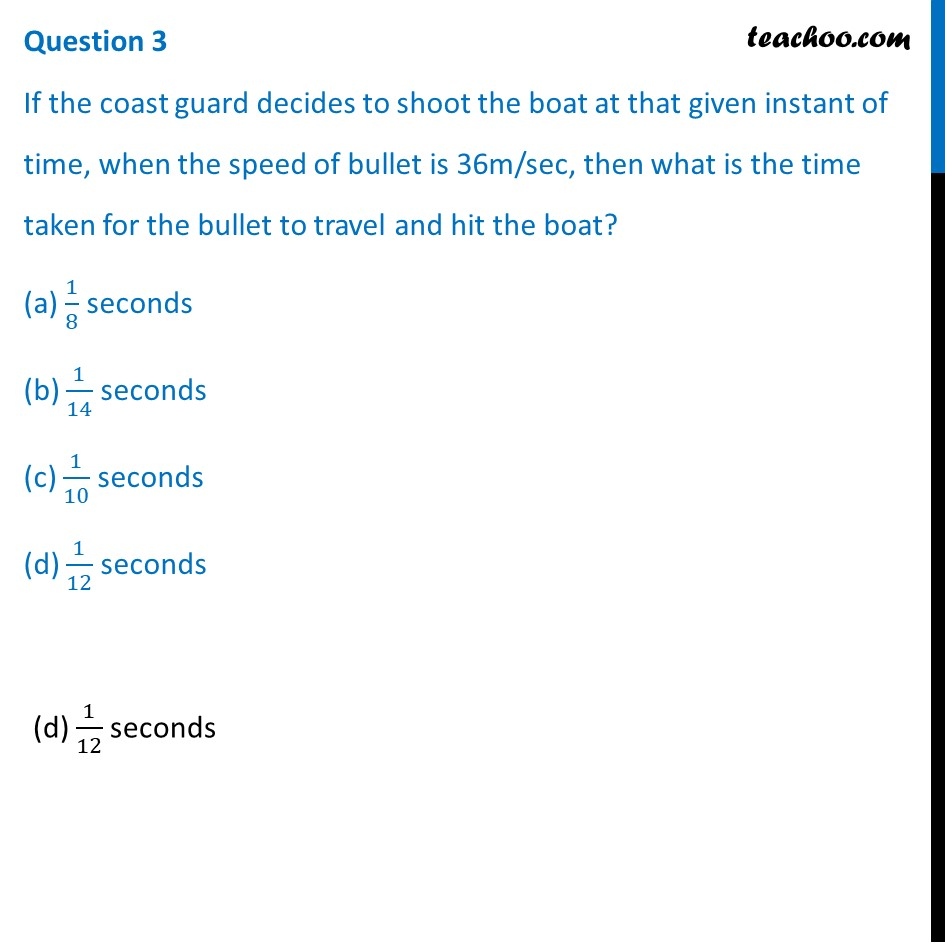## (d) (x - 1)/2=(y + 3)/(-1)=(z + 5)/2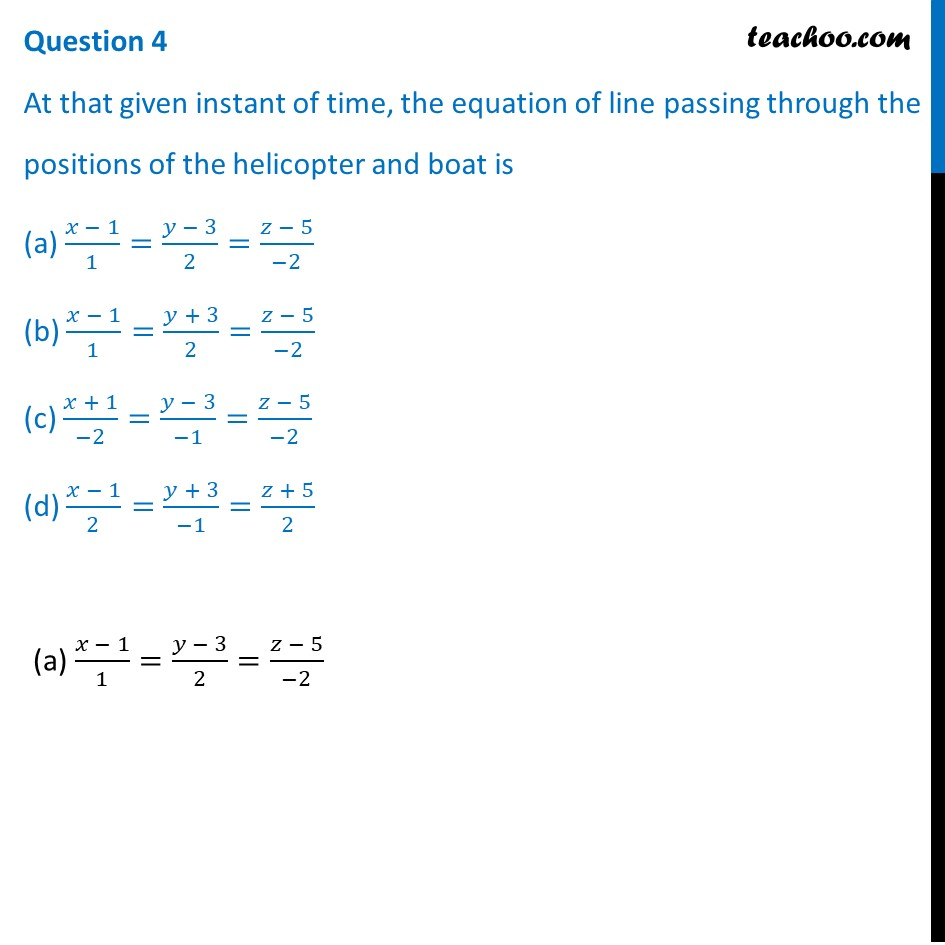## (d) none of the above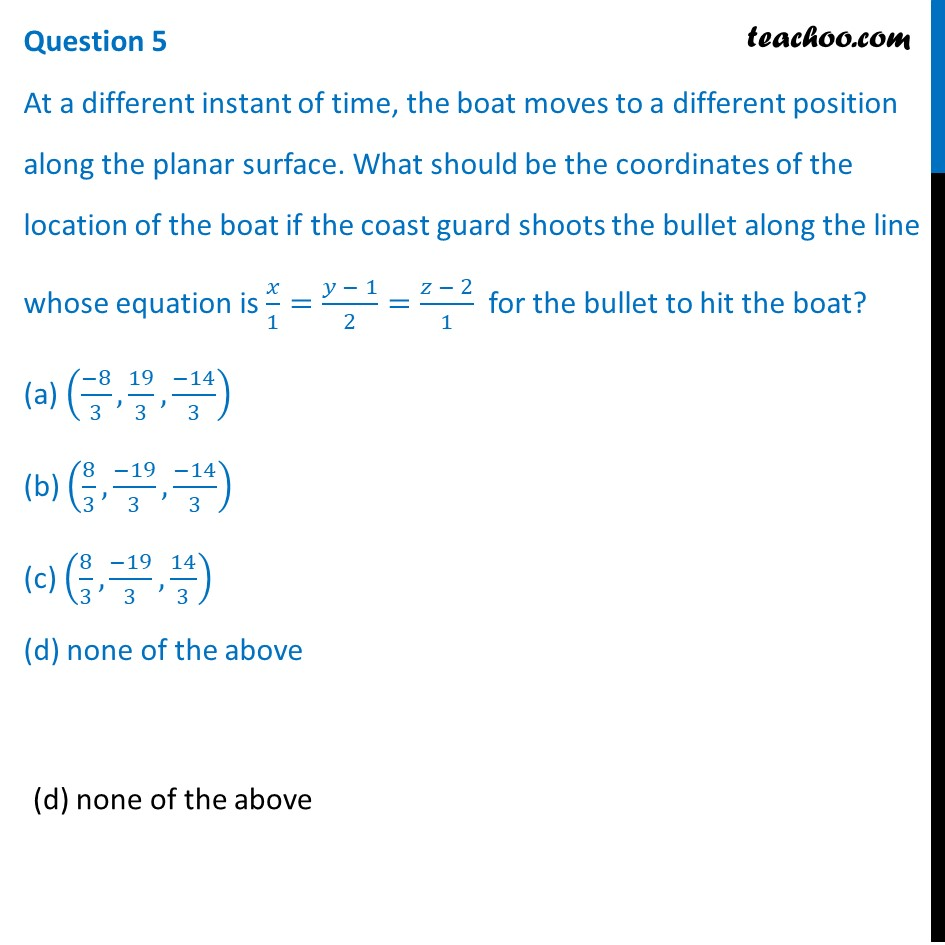1. Chapter 11 Class 12 Three Dimensional Geometry (Term 2)
2. Serial order wise
3. Case Based Questions (MCQ)

Transcript

Question The Indian coast guard, while patrolling, saw a suspicious boat with people. They were nowhere looking like fishermen. The coast guard were closely observing the movement of the boat for an opportunity to seize the boat. They observed that the boat is moving along a planar surface. At an instant of time, the coordinates of the position of the coast guard helicopter and the boat is (1, 3, 5) and (2, 5, 3) respectively. Based on the above answer the following:Question 1 If the line joining the positions of the helicopter and the boat is perpendicular to the plane in which the boat moves, then the equation of the plane is (a) –x + 2y – 2z = 6 (b) x + 2y + 2z = 6 (c) x + 2y – 2z = 6 (d) x – 2y – 2z = 6(c) x + 2y – 2z = 6 Question 2 If the coast guard decide to shoot the boat at that given instant of time, then what is the distance (in meters) that the bullet has to travel? (a) 5m (b) 3m (c) 6m (d) 4m(b) 3m Question 3 If the coast guard decides to shoot the boat at that given instant of time, when the speed of bullet is 36m/sec, then what is the time taken for the bullet to travel and hit the boat? (a) 1/8 seconds (b) 1/14 seconds (c) 1/10 seconds (d) 1/12 seconds (d) 1/12 seconds Question 4 At that given instant of time, the equation of line passing through the positions of the helicopter and boat is (a) (𝑥 − 1)/1=(𝑦 − 3)/2=(𝑧 − 5)/(−2) (b) (𝑥 − 1)/1=(𝑦 + 3)/2=(𝑧 − 5)/(−2) (c) (𝑥 + 1)/(−2)=(𝑦 − 3)/(−1)=(𝑧 − 5)/(−2) (d) (𝑥 − 1)/2=(𝑦 + 3)/(−1)=(𝑧 + 5)/2 (a) (𝑥 − 1)/1=(𝑦 − 3)/2=(𝑧 − 5)/(−2) Question 5 At a different instant of time, the boat moves to a different position along the planar surface. What should be the coordinates of the location of the boat if the coast guard shoots the bullet along the line whose equation is 𝑥/1=(𝑦 − 1)/2=(𝑧 − 2)/1 for the bullet to hit the boat? (a) ((−8)/3,19/3,(−14)/3) (b) (8/3,(−19)/3,(−14)/3) (c) (8/3,(−19)/3,14/3) (d) none of the above (d) none of the above

Case Based Questions (MCQ)